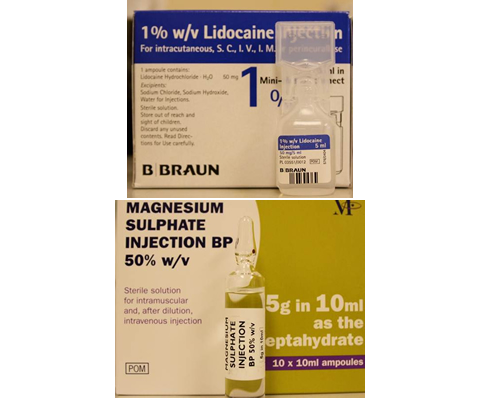## Concentration as %

%w/v stands for percentage weight in volume which is grams in 100 ml:

• 1% = 1 g in 100 ml ( =1000mg in 100ml = 10mg in 1 ml)
• 50% = 50 g in 100 ml (= 500 mg in 1 ml = 5 g in 10 ml)

To convert percent to milligram (mg):

Either multiply the percent strength by 10 or move the decimal point one space to the right. For example 1% lidocaine = 10 mg/ml

To convert milligram (mg) to percent:

Move the comma one space to the left. For example lignocaine 20 mg/ml = 2%